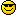# Sorry your browser is not supported!

You are using an outdated browser that does not support modern web technologies, in order to use this site please update to a new browser.

Browsers supported include Chrome, FireFox, Safari, Opera, Internet Explorer 10+ or Microsoft Edge.

### Code Snippets / [DBP] - Draw a line up to *but not past* a circle

Message
Posted: 13th Mar 2011 08:33 Edited at: 8th Oct 2011 00:28

errm, I'm no sure i could explain it better than running the program explains it.
Basically, it tries to draw a line from point1 to point2. if that line intersects the circle defined by point C and radius r, then the line is only drawn from point1 up to the point of intersection. This is *directional*, so drawing from point1 to point2 isn't the same as drawing from point2 to point1.

Posted: 13th Mar 2011 14:09
Pretty cool!ExoDev.Com - A Game Development Community Website! Featuring: XBOX360 CONTROLLER LIBRARY
Posted: 14th Mar 2011 11:29
Wonder why I can't the above to run, it compiles then falls over, the other demo you did of this works fine , so I was hoping to see what this does, but it won't let me, is it using any plug ins that not aware of?

Ho yes, running in windows 7, might have something to do with it.

Dark Physics makes any hot drink go cold.
Posted: 14th Mar 2011 11:35
OH! probably the lock/unlock pixels commands... they must have win7 issues. Remove 'em and it should run... maybe slowly though.

Posted: 14th Mar 2011 12:15
did the trick, that look great, could sort of use it to do letter glow like in one of the superman movies.

ran at a good speed with the lock unlock removed.

Dark Physics makes any hot drink go cold.
Posted: 14th Mar 2011 14:26
I can nearly get it to do what I am after, but I get a few pixles that do not turn black, run the code and you'll see what I doing.

Dark Physics makes any hot drink go cold.
Posted: 15th Mar 2011 04:28
Huh, I don't totally see what you're trying to do. I'll explain to you how I solved for the circleclip line funtion tho.

Basically, you have vectors P1 (draw from), P2 (draw to), and C (circle position), and scalar R (radius of the circle at position C).

This gives a parametric line:
Pt=(P2-P1)*t+P1

To solve for where the parametric lines intersect the circle, you solve for t, where the distance between Pt and C is R. In other words:

R=||Pt-C||=||(P2-P1)*t+P1-C||=sqrt(((P2.x-P1.x)*t+P1.x-C.x)^2+((P2.y-P1.y)*t+P1.y-C.y)^2)
that is, solve for t in the equation:
R^2=((P2.x-P1.x)*t+P1.x-C.x)^2+((P2.y-P1.y)*t+P1.y-C.y)^2

You end up with a quadratic polynomial looking like:
T^2*((P2.x-P1.x)^2+(P2.y-P1.y)^2 +
2*T*((P2.x-P1.x)*(P1.x-C.x)+(P2.y-P1.y)*(P1.y-C.y)) +
(P1.x-C.x)^2+(P1.y-C.y)^2-R^2

solving for T will either give you a complex answer if the line doesn't intersect with the circle. Otherwise, you get 2 possible values for T (or one double solution but whatevs). Take the smallest positive value of T, while restricting T to be on the interval [0,1]. Then draw the parametric line from 0 to T.